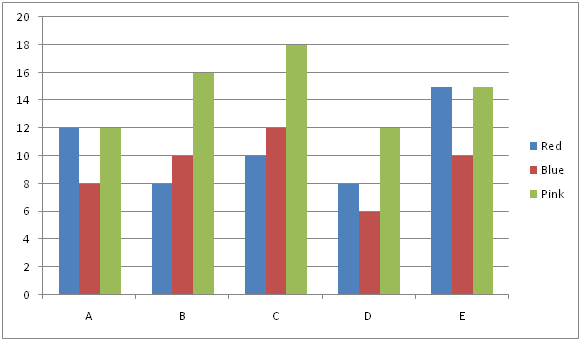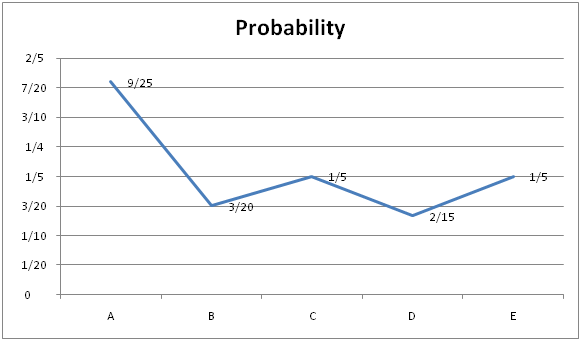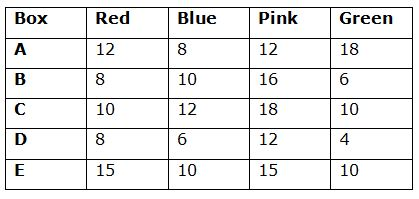# IBPS RRB PO Mains Quantitative Aptitude Questions 2019 (Day-04)

Dear Aspirants, Our IBPS Guide team is providing new series of Quantitative Aptitude Questions for IBPS RRB PO Mains 2019 so the aspirants can practice it on a daily basis. These questions are framed by our skilled experts after understanding your needs thoroughly. Aspirants can practice these new series questions daily to familiarize with the exact exam pattern and make your preparation effective.

Check here for IBPS RRB PO Mains Mock Test 2019

Check here for IBPS RRB Clerk Mock Test 2019

Check here for IBPS PO Exam Mock Test 2019

### Click Here to Subscribe Crack High Level Puzzles & Seating Arrangement Questions PDF 2019 Plan

[WpProQuiz 6851]

Directions (1 – 5): Study the following graph carefully and answer the given questions

The bar graph shows the no. of Red, Blue and Pink colour toys in a box A, B, C, D and EThe line graph shows the probability of a green colour toy taken from each boxes1) If 25% of red toys taken from box A and added to box E, then find the probability of taken two toys both are pink colour in box E

a) 98/1378

b) 105/1353

c) 105/1378

d) 102/1378

e) 115/1378

2) If the total no of red colour toys and pink colour toys from all the boxes (A, B, C, D and E) are taken out and filled in a new box P, then 2 toys taken out from box P find the probability of different colours

a) 3854/7875

b) 3869/7875

d) 3857/7875

e) 3814/7875

3) Four toys taken from box C, then find the probability of different colour

a) 116/2303

b) 226/2303

c) 256/2303

d) 216/2303

e) 96/2303

4) Total number of balls in box B and D together is approximately what percentage of the total number of balls in box C and E together?

a) 60

b) 50

c) 40

d) 80

e) None of these

5) Two balls taken randomly from box B. What is the probability of at least one pink colour ball?

a) 42/65

b) 41/65

c) 36/65

d) 38/65

e) 48/65

Directions (6 – 10): Each question contains a statement followed by Quantity I and Quantity II. Read the contents clearly and answer your questions accordingly.

6) Quantity I: 5, 8, 21, 80, 395, ?

Quantity II: 15, 40, 100, 230, 500, ?

a) Quantity I > Quantity II

b) Quantity I ≥ Quantity II

c) Quantity II > Quantity I

d) Quantity II ≥ Quantity I

e) Quantity I = Quantity II or Relation cannot be established

7) Quantity I: 6x2+11x-35=0

Quantity II: 2x2 – 40x + 200= 0

a) Quantity I > Quantity II

b) Quantity I ≥ Quantity II

c) Quantity II > Quantity I

d) Quantity II ≥ Quantity I

e) Quantity I = Quantity II or Relation cannot be established

8) Quantity I: Time taken by P to complete 1/5th of work if Q takes 6 days to complete 3/5th of work and together they take 5 days to complete 3/4th of work

Quantity II:  Time taken by a train to cross a platform of length 60 km given that it crosses a pole in two and a half hours running at 60 km/hr

a) Quantity I > Quantity II

b) Quantity I ≥ Quantity II

c) Quantity II > Quantity I

d) Quantity II ≥ Quantity I

e) Quantity I = Quantity II or Relation cannot be established

9) Quantity I: Age of M five years ago if 6 years hence ratio of age of M to N will be 14: 11, and 1 year ago the ratio was 7: 5.

Quantity II:  Average age of 2 students included in a group of 5 students with average age 18 years given that addition of these 2 students in group makes average increase by 1.

a) Quantity I > Quantity II

b) Quantity I ≥ Quantity II

c) Quantity II > Quantity I

d) Quantity II ≥ Quantity I

e) Quantity I = Quantity II or Relation cannot be established

10) A shopkeeper gets a discount of 20% on an article and sells it at 10% profit.

Quantity I: Labeled price as a percent of cost price.

Quantity II:  Profit percent if discount given is 60%

a) Quantity I > Quantity II

b) Quantity I ≥ Quantity II

c) Quantity II > Quantity I

d) Quantity II ≥ Quantity I

e) Quantity I = Quantity II or Relation cannot be established

Directions (1 – 5):

Box A:

Let us take the no. of green colour toys be x

Probability = xc1/(32+x)c1

9/25 = x/(32+x)

32*9 +9x = 25x

16x = 32*9=> x= 18 green colour toys

Box B:

Let us take the no. of green colour toys be x

Probability = xc1/(34+x)c1

3/20 = x/(34+x)

3*34+ 3x = 20x

=>3*34= 17x

X= 6 green colour toys

Box C:

Let us take the no. of green colour toys be x

Probability = xc1/(40+x)c1

1/5 = x/(40+x)

=>40+x= 5x

=>4x=40 =>x= 10 green colour toys

Box D:

Let us take the no. of green colour toys be x

Probability = xc1/(26+x)c1

2/15 = x/(26+x)

52+2x= 15x

=>13x= 52

=> x= 4 green colour toys

Box E:

Let us take the no. of green colour toys be x

Probability = xc1/(40+x)c1

1/5 = x/(40+x)

=>40+x= 5x

=>4x=40 =>x= 10 green colour toysTotal number of balls in box E = [(12*25/100)+15] +10+15+10

= 18+10+15+10

= 53

Required probability = 15c2/53c2 = (15*14)/(53*52)

= 105/1378

Total number of red colour boys in box P = (12+8+10+8+15) = 53

Total number of pink colour balls in box P = (12+16+18+12+15) = 73

Required probability = (53*73)/(53+73)c2

= 53*73/(126*125/1*2) = 3869/7875

Required probability = (10*12*18*10)/50c4

= (10*12*18*10)/(50*49*48*47/1*2*3*4)

= (24*9)/(49*47)

= 216/2303

Required percentage

= [(8+10+16+6+8+6+12+4)/(10+12+18+10+15+10+15+10)]*100

= 70/100 * 100 = 70%

Required probability = 1- 24c2/40c2

= 1 – (24*23/40*39)

= 1- 23/65

= 42/65

Direction (6-10) :

Quantity I:

5*2-2 = 8

8*3-3 = 21

21*4-4 = 80

80*5-5 = 395

395*6-6 = 2364

Quantity II:

15*2+10 = 40

40*2+20 = 100

100*2+30 =230

230*2+40 =500

500*2+50 =1050

Quantity I > Quantity II

Quantity I: 6x2 + 11x – 35 = 0

6x2 – 21x + 10x – 35 = 0

3x (2x – 7) + 5(2x – 7) = 0

(2x – 7) + (3x + 5) = 0

X = 7/2, -5/3

Quantity II: 2x2 – 40x + 200= 0

x2– 20x + 100 = 0

x (x – 10) – 10 (x – 10) = 0

(x – 10) (x – 10) = 0

X = 10, 10

Quantity I < Quantity II

Quantity I: P and Q completes 3/4th work in 5 days, so complete 1 work in 4/3 * 5 = 20/3 days

Q complete 3/5 work in 6 days, so complete work in 5/3 * 6 = 10 days

So in 1 day P completes = 3/20 – 1/10 = 1/20

So to complete 1/5th work = 1/5 * 20 = 4 days

Quantity II: length of train = 2.5 * 60 = 150 km

So time taken to cross platform of length 60 km with speed 60 km/hr

= (150+60)/60 = 3.5hrs

Hence, Quantity I >Quantity II

Quantity I: (M+6)/(N+6) = 14/11

And (M-1)/(N-1) = 7/5

Solve the equations, M = 22, so 5 years ago = 22 – 5 = 17 years

Quantity II: Total age of 2 students added to group = 18*2 + 7*1 = 43

So their average age = 43/2 = 21.5 years

Hence Quantity I < Quantity II

Quantity I: MP = (100+10)/(100-20) * CP = 11/8 of CP

So required % = MP/CP * 100 = (11CP/8)/CP * 100 = 137.5%

Quantity II: % profit = (100+10) [(100-10)/(100-60)] – 100 = 590/4 = 147.5%

Hence Quantity I < Quantity II